# Help with resistance of meterials

#### Guest3123

Joined Oct 28, 2014
404
So.. I understand that Copper, SIlver and Graphene are the most conductive materials on the face of the planet.

p (Ω•m) 20 ºC

What does the above thing mean? It's to do with resistance... right? I don't understand what the p symbol means. Ohms x meter, at 20ºC (68ºF)

I also can't find the ALT code for the p symbol. So I wrote it as a lowercase p instead.

Understandably.. Copper has a Resistance of 0.0000000168 Ω/m,

At what Length, Width, Height does copper have a resistance of 0.0000000168 Ω/m ?

Understandably.. Just for an example of my current understanding of resistance of copper. A piece of 12 Gauge Copper, at 1 Foot. has a resistance of 0.001588Ω That's understandable, and I can work with that. But the chart below is mostly foreign to me.

Also, 12 Gauge Copper wire has a thickness of 0.08080", that too is understandable.Image Credit to WIkipedia. Modified with Adobe PhotoShop CS5.1

https://en.wikipedia.org/wiki/Electrical_resistivity_and_conductivity

I would like to learn a little bit about that specfic part, and then if someone wants to talk a little bit about the rest, which would be the conductivity, etc. that would be cool too. etc.

#### Papabravo

Joined Feb 24, 2006
20,385
The symbol you are seeking is the greek letter rho --> ρ
Here is the unicode datasheet
http://www.fileformat.info/info/unicode/char/03c1/index.htm

Read the article and track the units

https://en.wikipedia.org/wiki/Electrical_resistivity_and_conductivity

ρ has units of ohms⋅meters because the definition includes an area divided by a length. Resistivity does not depend on the geometry of the sample; it depends only on the intrinsic property of the material. It also seems like if you know the resistivity you can find the resistance of a sample if you know the cross sectional area and the length. You should be able to figure out how that would be done -- right?

•Guest3123

#### Guest3123

Joined Oct 28, 2014
404
The symbol you are seeking is the greek letter rho --> ρ
Here is the unicode datasheet
http://www.fileformat.info/info/unicode/char/03c1/index.htm

Read the article and track the units

https://en.wikipedia.org/wiki/Electrical_resistivity_and_conductivity

ρ has units of ohms⋅meters because the definition includes an area divided by a length. Resistivity does not depend on the geometry of the sample; it depends only on the intrinsic property of the material. It also seems like if you know the resistivity you can find the resistance of a sample if you know the cross sectional area and the length. You should be able to figure out how that would be done -- right?

Ok man.. I'm willing, and I'm trying. I would really like to learn how to do this.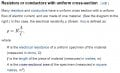So copper has ρ (Ω·m) of 0.0000000168Ω/m

A 12 gauge piece of copper wire has a length of 1 ft. (0.3048 meters), diameter of 0.08080" (0.02462784m),
To Convert Diameter to area is.. 0.02462784

π•d² / 4 = Area for a specific diameter.

First.. I'll find out what the diameter is squared. 0.02462784² = 0.0006065305030656
So 3.1415926535 x 0.0006065305030656 = 0.0019054717725545481885696 / 4 = 0.0004763679431386370471424 given in meters.

So the area is 0.0004763679431386370471424 Sq. Meters.
The Length, in meters is 0.3048 Meters. (1 Foot).

R is the electrical resistance of a uniform specimen of the material (measured in ohms, Ω)is the length of the piece of material (measured in metres, m)
A is the cross-sectional area of the specimen (measured in square metres, m2).= 0.3048 Meters
A = 0.0004763679431386370471424 Sq. Meters.
R = IDK.. That's what I'm trying to figure out.. wth..?

The Electrical resistivity is equal to (Rho ρ).

So ρ = Ω • A /Is R or Ω's = 0.0000000168 ?
So is it.. ρ = 0.0000000168Ω • 0.0004763679431386370471424 Sq. Meters / 0.3048 Meters.

I don't know how to do this..

#### Guest3123

Joined Oct 28, 2014
404
Ok.. Just watched a video on how to find the cross sectional area..

So to find the Cross sectional area, given the radius..

A = π•R²

So..... the diameter of 12 gauge copper wire is.. 0.08080" or 0.02462784 meters.

So divide that by two to get the radius.. 0.02462784 / 2 = 0.01231392 is the radius in meters for 12 gauge copper wire.

0.01231392² = 0.0001516326257664 Meters.

3.1415926535 • 0.0001516326257664 = 0.0004778842693963010471424

So the Cross sectional area is actually.. 0.0004778842693963010471424 Sq. Meters.

I'm actually still lost.. Because I don't know what R is.. R being the Resistance in Ohm's.. which is what I'm trying to figure out.

#### Papabravo

Joined Feb 24, 2006
20,385
OK you have the basic definition of ρ. You know what the units of each of the quantities is:
1. ρ is Ω⋅m
2. R is Ω
3. l is m
4. A is m⋅m, or m^2
Multiply both sides of the equation by the ratio of l/A and you get:

$$\frac{l}{A} \cdot \rho = R \cdot \frac{A}{l} \cdot \frac{l}{A}= R$$

Lets check the units of that result

$$(\text meters / meters^2)\;\times\;\text ohms\cdot\text meters =\;\text ohms$$

$$1.68 \times 10^{-8} \text is resistivity \rho \text , in ohms\cdot meters$$

Multiply ρ by l over A to get resistance R

NB: The units of ρ are not, REPEAT NOT, Ω/m. Sloppy tracking of units is the road to perdition. Know it, learn it, believe it!

Last edited:
•Guest3123

#### Guest3123

Joined Oct 28, 2014
404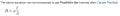R = ρ•/ A

So !!!= 0.3048

A = 0.0001516326257664 Sq. Meters (Cross Sectional Area)
ρ = 0.0000000168/ A = 0.3048 / 0.0001516326257664 = 2010.1214923862387018307350342113

R = 0.0000000168 • 2010.1214923862387018307350342113
R = 3.377004107208881019075634857475e-5 (0.00003377004107208881019075634857475)

So... !!!

ρ = R • A /So that's..
ρ = 0.00003377004107208881019075634857475R • (0.0001516326257664 A / 0.30480.0001516326257664 A / 0.3048 = 0.000497482368

ρ = 0.00003377004107208881019075634857475R • 0.000497482368 = 1.6799999999999999999999999997637e-8
p = 0.000000016799999999999999999999999997637

Umm... wtf..? Isn't that what Rho is for Copper..? But I used a set length and gauge of copper wire..?

I'm so lost...

#### Guest3123

Joined Oct 28, 2014
404
OK you have the basic definition of ρ. You know what the units of each of the quantities is:
1. ρ is Ω⋅m
2. R is Ω
3. l is m
4. A is m⋅m, or m^2
Multiply both sides of the equation by the ratio of l/A and you get:

$$\frac{l}{A} \cdot \rho = R \cdot \frac{A}{l} \cdot \frac{l}{A}= R$$

Lets check the units of that result

$$(\text meters / meters^2)\cdot \text ohms\cdot\text meters =\text ohms$$

$$1.68 \times 10^{-8} \text is resistivity \rho \text , in ohms\cdot meters$$

Multiply ρ by l over A to get resistance R

NB: The units of ρ are not REPEAT NOT, Ω/m. Sloppy tracking of units is the road to perdition. Know it, learn it, believe it!

Ok.. Yeah, your right.. I was a little sloppy.
So in order to get R, I need to multiply p • (I/A).

Got it.. So let me try.

12 Gauge Copper Wire= 0.3048
A = 0.0001516326257664 Sq. Meters (Cross Sectional Area)
ρ = 0.0000000168

in order to get R, I need to multiply p • (I/A).
0.0000000168p • (0.3048/ 0.0001516326257664 A)

First things first. (0.3048/ 0.0001516326257664 A) = 2010.1214923862387018307350342113
0.0000000168 p • 2010.1214923862387018307350342113 = 3.377004107208881019075634857475e-5

Or... 0.00003377004107208881019075634857475 Ω's ?

So a 1 Foot piece of 12 Gauge Copper Wire has a resistance of 0.00003377004107208881019075634857475 Ohm's ?

That doesn't seem right.. Idk what I did wrong..

0.0000000168 * 3.28084 Meters in a Foot = 0.0001107941015509518520262410586424

Geesh.. 0.001588Ω is what they give me online for Resistance for 12 gauge copper wire.

0.001588000 (Online Resources)

Last edited:

#### HitEmTrue

Joined Jan 25, 2016
32
You haven't calculated the diameter of the wire correctly, yet.

The resistance is a very small number. Instead of typing out a bunch of zeros after the decimal, you should use scientific notation.

< edit >
I see that you were using scientific notation in parts of your reply. Have you learned about significant digits? You are messing around with with too many digits.

•Guest3123

#### Guest3123

Joined Oct 28, 2014
404
....

Last edited:

#### Guest3123

Joined Oct 28, 2014
404
You haven't calculated the diameter of the wire correctly, yet.

The resistance is a very small number. Instead of typing out a bunch of zeros after the decimal, you should use scientific notation.

< edit >
I see that you were using scientific notation in parts of your reply. Have you learned about significant digits? You are messing around with with too many digits.
Ok man.. I'll learn how to do Scientific notation on the calculator.

I know that 1.68e-8 is 0.0000000168, but I'll learn how to use what seems like smaller simplier numbers, but I'll write in scientific notation instead of writing out the whole number, lol.. Ok.. off to learn how to do it on the calculator.

#### Jony130

Joined Feb 17, 2009
5,457
•Guest3123

#### HitEmTrue

Joined Jan 25, 2016
32
I like to see the whole number.. 0.0000000168 is what 1.68e -8 is. It's the same thing.

It's 0.0808" is the diameter of the 12 gauge copper wire. According to Google.
0.0808" is 0.00205232 Meters Diameter. According to Google.
In some previous posts you were using 0.02462784m as the diameter. 0.00205232m is the correct diameter.

One disadvantage of NOT using scientific notation is that it is easier to make mistakes when doing calculations...

Last edited:
•Guest3123

#### Papabravo

Joined Feb 24, 2006
20,385
In order to duplicate various results you need to know what the original assumptions were. When dealing with ratios of large and small numbers small errors can become significant very quickly. Instead of looking for correspondence of result look for how close you can get. I'm generally happy if my answer has the same order of magnitude. LOL

To find the resitance of 1 foot of #12 AWG copper wire:
I compute the cross sectional area of #12 AWG copper to be: $$3.31 \times 10^{-6} \text meters^2$$
I think that agrees with your result to 3 significant figures

$$\frac {(1.68 \times 10^{-8})(0.3048)}{3.31 \times 10^{-6}} = 1.547 \times 10^{-3}\;\Omega$$

Google lists the ohms per 1000 feet of #12 AWG copper as 1.588 ohms, so our answer is low by 2.6% which I actually think is pretty good agreement. I actually worked this out on my Eugene Dietzen Log-Log Duplex Deci-trig Slide Rule -- because I can. Would the answer improve with more significant figures -- maybe a little bit. The problem is we don't really know how the figure in the table was arrived at. Was it calculated or measured? If it was measured how close was the diameter and length to the assumed values. We just don't know.

https://en.wikipedia.org/wiki/Slide_rule

Last edited:

#### Kermit2

Joined Feb 5, 2010
4,162
Values of resistance for copper are all over the place. Some sites using figures published in the 20's. Modern foundry practice and electrolytic refining have produced purer copper since those days and an old work around was inclusion of copper catagories with 101% conductivity or even higher. That was ridiculous and was changed again, but older sources of information still show it. Web sites seem to take whatever value they find first and run with it.

#### Guest3123

Joined Oct 28, 2014
404
12 gauge copper wire = 3.308 mm^2 --->3.308×10^-6 square meters
http://www.wolframalpha.com/input/?i=12+gauge&a=*C.12+gauge-_*WireGauge-
1 ft = 0.3048 meters
ρ - 1.68 x 10^-8 Ω*m
R = 1.68 x 10^-8 Ω*m *(0.3048 meters/ 3.308×10^-6 square meters) = 0.0015458Ω
http://www.wolframalpha.com/input/?i=R+=+1.68+x+10^-8+Ω*m+*(0.3048+meters/+3.308×10^-6+square+meters)
Ok.. So I did a little more research, and tried again.

(Lazy) : http://www.da-share.com/calculators/wire-cross-section-area/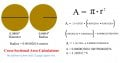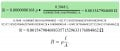By god I think I got it now.. thanks so much for all the help.

Now.. to calculate for Silver.. Someone might say.. silver? where do you get silver wire from..?

To prove that I have this stuff down pat, I'll go ahead, and do it for Silver..As if the above drawing and stuff in the above uploaded images of my work, and understanding that I drew up in InkScape wasn't enough.. I would like to do it anyways, for this post.

#### Guest3123

Joined Oct 28, 2014
404
I would really like to know how to back out of a reply.. I really would.. I searched all the features to the freaking reply thing, and I just can't figure out how the heck to back out. this is the millionth time I've tried to get out of a reply.. and even if I log out, and come back the reply is still in memory..

#### Papabravo

Joined Feb 24, 2006
20,385
Line 3 of your area calculation has an extra 3 in it. You wrote A = 3.3308 E-6, but it should be 3.308 E-6. You did correct it in the final calculation however. This should be an object lesson in trying to carry too many significant digits. Take it from an engineering career that lasted half a century, the extra digits are asking for trouble with back of the envelope calculations.

•Guest3123

#### Guest3123

Joined Oct 28, 2014
404
In order to duplicate various results you need to know what the original assumptions were. When dealing with ratios of large and small numbers small errors can become significant very quickly. Instead of looking for correspondence of result look for how close you can get. I'm generally happy if my answer has the same order of magnitude. LOL

To find the resitance of 1 foot of #12 AWG copper wire:
I compute the cross sectional area of #12 AWG copper to be: $$3.31 \times 10^{-6} \text meters^2$$
I think that agrees with your result to 3 significant figures

$$\frac {(1.68 \times 10^{-8})(0.3048)}{3.31 \times 10^{-6}} = 1.547 \times 10^{-3}\;\Omega$$

Google lists the ohms per 1000 feet of #12 AWG copper as 1.588 ohms, so our answer is low by 2.6% which I actually think is pretty good agreement. I actually worked this out on my Eugene Dietzen Log-Log Duplex Deci-trig Slide Rule -- because I can. Would the answer improve with more significant figures -- maybe a little bit. The problem is we don't really know how the figure in the table was arrived at. Was it calculated or measured? If it was measured how close was the diameter and length to the assumed values. We just don't know.

https://en.wikipedia.org/wiki/Slide_rule

How is it off by 2.6%..? We were given 1.68E-6 by Wikipedia.. how can I find better information that that then.. I want to get a more accurate answer. Also.. did you see what I posted..? lol.

#### Papabravo

Joined Feb 24, 2006
20,385
How is it off by 2.6%..? We were given 1.68E-6 by Wikipedia.. how can I find better information that that then.. I want to get a more accurate answer. Also.. did you see what I posted..? lol.
$$\frac{1.588 - 1.547}{1.588}= .0258\dots\;\approx\;2.6%$$

I can't say where the source of the error is, but I can say that the CRC Hnadbook of Chemistry and Physics might provide better information.

http://www.amazon.com/Handbook-Chem...eywords=CRC+Handbook+of+chemistry+and+Physics

A steal at \$0.50 plus shipping

•Guest3123

#### Guest3123

Joined Oct 28, 2014
404
Doesn't seem like much of a difference, but here it is.. .999 Silver (12 Gauge, SOLID).

0.00154790468833571529633176884862 Ω/FT (12 Gauge Copper, 0.0808" (2.05232mm))
0.00152732020883115415864033638192 Ω/FT (12 Gauge Silver, 0.07913" (2.01mm))
Yeah.. the Silver wire from RioGrande is actually a bit smaller in diameter.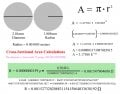I would like to know where I can find better resistance values, other than the crappy 1.68 or 1.59 from Wikipedia.. they're off by 2.6%.